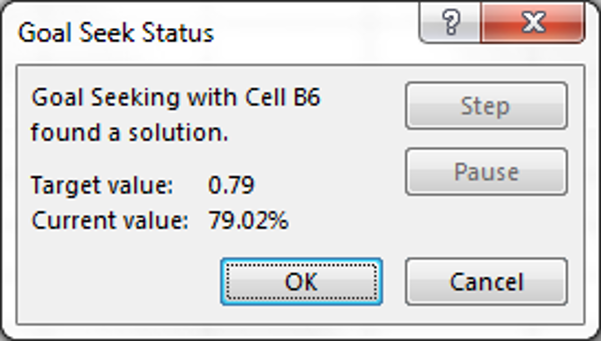top of pageSearch

# Goal Seek

MS Excel 2016 contains a tool to help you get the results you need from formulas. Goal Seek will automatically find one of values that must be entered in a formula in order to get a result from the formula that you designate.

Take this example working with the demonstration of the weighted average formula discussed in last night's tip. Let's say for the sake of argument that we didn't know the number of loans in deal Beta, but we did know that the weighted average of the LTV ratios in the two deals was 79%. We could find the number of loans in deal Beta by setting up the data and formula as we did to get the weighted average when the average of each set and the number of values in each set was known. With the formula entered in cell B6 selected, on the Data tab, browse to 'What-If Analysis' in the Forecast section on the right, and select 'Goal Seek' in the drop down menu.The set cell with the formula is B6. We enter the value we need and then the cell that has to be changed to get that value.Goal Seek will run a calculation that will try to come as close to the requested result as possible.In this case the result isn't very satisfying. We don't get a whole number of loans for deal Beta and the weighted average given is not exactly 79%. We can try to get a better result by making a change to the Formula options.Set the 'Minimum Change' value to a very low decimal number. E.g., "0.00000000001".

Using the Goal Seek tool again, should give a better result.bottom of page